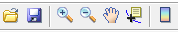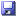MRI Graph Analysis

GUIMRIGraphAnalysis is a graphical user interface that allows the user to define the parameters to create the connectivity matrices to analyze MRI data, while simultaneously visualizing the resulting weighted or binary matrices. Binary connectivity matrices can be visualized as a function of density or threshold. The user can also define a community structure and restrict the analysis to a subset of brain regions. A list of the measures available for calculation is shown at the bottom of the interface. The MRI graph analysis can be saved in a file *.mga for future use within BRAPH; it also can be exported in xml format for use within other programs.Figure 1: Screenshot of GUIMRIGraphAnalysis. On the top, there are the menu and the toolbar; in the middle there are the cohort panel and the graph analysis panel (on the left), and the main panel (on the right); on the bottom, there is the measures panel.

The layout of GUIMRICohort is shown in figure 1. It is composed of six main work areas:

• Menu permits one to access the basic functionalities of GUIMRIGraphAnalysis, including loading and saving an MRI graph analysis.
• Toolbar gives direct access to some of the most commonly employed functionalities, in particular loading and saving an MRI graph analysis as well as manipulating the graphic representations of the connectivity matrices.
• Cohort panel permits one to select an MRI cohort for the graph analysis or, if already selected, to view the cohort properties in GUIMRICohort.
• Graph analysis panel permits one to choose the properties of the graph analysis, to set a community structure, and to choose whether to perform the analysis on a subgraph.
• Main panel visualizes the connectivity matrix that will be used for the analysis.
• Measures panel shows the available measures for each type of graph (binary or weighted).

Example data and tutorial videos can be found on http://braph.org/videos/mri/mri-graph-analysis/.

Getting Started

As a first example of the use of GUIMRIGraphAnalysis, we will proceed to import the MRI cohort stored in the my_cohort.mc file. Then, we will define a binary undirected graph analysis with fixed density and positive Pearson correlation coefficients. We will further specify a dynamic community structure calculated with the Louvain algorithm by using the subject group gr1_MRI. Finally, we will choose to perform the analysis on the full connectivity matrix and save it as a *.mga file.

1. Push Select Cohort to select an MRI cohort as shown in figure 2. The cohort must be in *.mc format. After you select the file, the cohort panel is updated to show the properties of the cohort. The select button state is changed to View Cohort; pushing this button opens the uploaded cohort in GUIMRICohort with restricted access (i.e. no further changes to the cohort are allowed).Figure 2: Importing an MRI cohort from a *.mc file into GUIMRIGraphAnalysis.

2. Select BUD from the ‘Graph’ popup menu in the graph analysis panel, Pearson from the ‘Correlation’ popup menu, and zero from the ‘Negative corrs.’ popup menu. With these settings, the connectivity matrix will be calculated using Pearson correlation coefficients where all negative coefficient are set to zero; this matrix will then be binarized at a fixed density.A number of settings are available to create different types of graph analyses.
More details about how these options affect the graphs can be found in the chapter ‘Brain Graphs’.
These options are accessible through the popup menus in the graph analysis panel:

• Graph sets the type of graph to be analyzed:
WU analyzes weighted undirected graphs.
BUT analyzes binary undirected graphs, i.e. graphs whose connectivity matrices are binarized by specifying the threshold.
BUD analyzes binary undirected graphs, i.e. graphs whose connectivity matrices are binarized by specifying the density.
• Correlation sets the correlation used to calculate connectivity
matrix coefficients:
Pearson is the Pearson product-moment correlation coefficient.
Spearman is the Spearman rank correlation coefficient.
Kendall is the Kendall rank correlation coefficient.
partial Pearson is the partial Pearson correlation coefficient.
partial Spearman is the partial Spearman correlation coefficient.
• Negative corrs. sets how to deal with the negative correlation
coefficients:
zero sets all negative correlation coefficients to zero.
none leaves all negative correlation coefficients as as they are.
abs replaces all negative correlation coefficients with their absolute values.
3. Push Edit in the panel ‘Community structure’ to define a community structure. This opens a new interface where the parameters for the calculation of the community structure can be set. Check the Dynamic structure checkbox to define a dynamic structure, choose the Louvain algorithm and select the group group1 from the popup menu, as shown in figure 3.Figure 3: Interface to define a community structure for the graph analysis.

The community structure interface consists of five main working areas as shown in figure 3:

• Menu permits one to generate the brain view of the community structure. This can be customized using the standard MatLab plotting tools. The figure can then be exported in several standard graphic formats.
• Toolbar gives direct access to various brain views and allows the user to choose how to represent the brain regions (spheres, symbols, or labels).
• Brain view panel visualizes the community structure on a brain surface. Different modules are represented with different colors.
• Parameter panel allows one to choose the parameters for the calculation of the community structure. The following parameters can be specified:

Fixed structure fixes the community structure. The same structure will be used throughout the analysis.

Dynamic structure creates a dynamic structure with the specified parameters. The structure will be recalculated with the selected parameters whenever needed throughout the analysis.

Louvain algorithm calculates the structure using the Louvain algorithm.

– Newman algorithm calculates the structure using the Newman algorithm.

Gamma sets the parameter$\gamma>0$ determining the resolution of the algorithm. The default setting is$\gamma = 1$. Larger values ($\gamma>1$) lead to more modules and smaller values ($0<\gamma<1$) to less modules.

Group permits one to choose the group whose data serve as basis for the community structure calculation.

The structure is calculated by pushing Calculate and reset by pushing Reset. Once all parameters are chosen, the structure can be set by pushing Set.

Alternatively, one can choose to perform the graph analysis with the default community structure by pushing Default. The default structure is a dynamic structure calculated with the Louvain algorithm with$\gamma=1$.

4. To start the graph analysis on the full connectivity matrix, push Start analysis. This opens a new interface, GUIMRIGraphAnalysisBUD, which allows one to calculate and visualize the graph measures. The details of this interface are discussed in chapter ‘MRI Graph Analysis BUD’. After this, the parameters of the analysis become fixed and, if any change is needed, a new graph analysis with different parameters should be created.Figure 4: Interface to determine a subgraph on which to perform the graph analysis.

5. The analysis can be performed only on a subset of brain regions. To do this, push Subgraph analysis.This opens a new interface, shown in figure 4, with five main working areas:
• Menu permits one to generate the subgraph brain view, which can be customized using the standard MatLab plotting tools. The figure can then be exported in several standard graphic formats.
• Toolbar gives direct access to various brain views and allows the user to choose how to represent the brain regions (spheres, symbols, or labels).
• Table panel shows all brain regions. If the checkbox next to a brain region is checked, the region is included into the subgraph.
• Brain view panel permits one to visualize the subgraph on a brain surface. The included brain regions are highlighted with an orange color.
• Control panel allows one to choose the parameters for the calculation of the subgraph analysis as follows: (1) One of the modules from a previously calculated community structure can be selected as subgraph from the popup menu on the right. (2) Set sets the subgraph that will be used in the analysis. (3) Start analysis starts the analysis by opening a new interface, GUIMRIGraphAnalysisBUD. The analysis can be performed only after the subgraph has been set.
6. Select File$\rightarrow$ Save to save the MRI graph analysis as a *.mga file; alternatively you can also use the shortcut Ctrl+S or the Save icon on the toolbar.
7. Select File$\rightarrow$ Open to open an MRI graph analysis previously saved with GUIMRIGraphAnalysis; alternatively you can also use the shortcut Ctrl+O or the Open icon on the toolbar. Opening a *.mga file with with GUIMRIGraphAnalysis interface opens a new interface (i.e. GUIMRIGraphAnalysisWU, GUIMRIGraphAnalysisBUT, or GUIMRIGraphAnalysisBUD) depending on the type of analysis specified (weighted undirected, binary undirected fixed threshold, or binary undirected fixed density, respectively).

Main panel

The main panel allows one to visualize the connectivity matrix based on the parameters specified by the set of checkboxes on the right (figure 1). The available options are:

• Group selects the group whose connectivity matrix to show.
• Weighted correlation matrix, if checked, visualizes the correlation coefficients between any two brain regions: warmer colors denote higher coefficients.
• Histogram shows the distribution of the correlation coefficients.
• Binary correlation matrix (set density) shows the binarized connectivity matrix at the set density (text field and slider below).
• Binary correlation matrix (set threshold) shows the binarized connectivity matrix at the set threshold (text field and slider below).
• Rearrange to reflect community structure rearranges the rows and columns of the connectivity matrix to reflect the community structures (i.e. keeping together regions belonging to the same module).
• Divide communities draws lines (squares around each module) to emphasize the division of the brain into different modules. This option is available only if the matrix has been previously rearranged to reflect the community structure by selecting the option Rearrange to reflect community structure.

Measures panel
The measures panel lists the measures (together with a short description)
that are available for calculation given the kind of graph that
has been selected (WU, BUT, BUD).

File provides various options for importing and saving an MRI graph analysis:

• File$\to$ Open (Ctrl+O) opens a popup window to load an MRI graph analysis saved in *.mga format.
• File$\to$ Close (Ctrl+C) closes the GUIMRIGraphAnalysis.
• File$\to$ Save (Ctrl+S) saves the MRI current graph analysis in *.mga format.
• File$\to$ Save as opens a popup window to save the current MRI graph analysis in *.mga format possibly in a different file.
• File$\to$ Import (xml) imports an MRI graph analysis from an xml file.
• File$\to$ Export (xml) exports the current MRI graph analysis to an xml file.

Brain View$\to$ Generate figure (Ctrl+F) generates a figure that can be customized using the standard MatLab plotting tools. The figure can then be exported in several standard graphic formats.
About$\to$ About provides information about the current version of GUIMRIGraphAnalysis and BRAPH.

Toolbar

The toolbar provides different options to open and save the MRI graph analysis as well as to visualize the connectivity matrix. It is shown in figure 5.Figure 5: GUIMRIGraphAnalysis toolbar.

Open and save commands

These commands allow the user to open and save an MRI graph analysis in the *.mga format. These are equivalent to the open and save menu options in the File menu.opens a popup window to load an MRI graph analysis saved in *.mga format.saves the current MRI graph analysis in *.mga format.

Visualization commands

These commands allow the user to control the visualization of the graphical representations of the connectivity matrix.zooms in image.zooms out image.drags image.shows/hides data cursor.shows the colorbar.

Watch also:

Footnotes and references

1. ^ Not all measures can be calculated in the presence of negative correlation coefficients.